# Logical Functoids Reference

Use Logical functoids to perform a variety of logical operations, often controlling whether a particular element or attribute is created in an output instance message.

Important

Because Microsoft BizTalk Server uses the underlying functionality of the .NET Framework, the results produced by some of the Logical functoids may vary from the equivalent functoids in earlier versions of BizTalk Server. For example, Logical functoids are case-sensitive when comparing two strings: "Abc" and "abc" are not equal. The exception to this rule is when Logical functoids compare strings that represent the Boolean values True and False:"True" and "true" are equal. You should test your maps thoroughly to ensure that you are getting the results you expect.

Unless otherwise noted, Logical functoids always output a Boolean value, either True or False. This means that you cannot use the output of a Logical functoid as the input to a functoid that is expecting a string, such as the Uppercase functoid, hoping to yield an output string like "TRUE". In other words, you should not connect the output of a logical functoid to a node and expect that field to be populated with a string value of "TRUE" or "FALSE" or variant ("True", "true", "False", "false", etc).

On the other hand, Logical functoids do accept a few different data types (Boolean values, strings, and numbers) as input values. They use standard semantics to interpret and compare such values. The best practice is to pass two parameters of the same data type, which results in the following actions:

• When both input parameters are Boolean values, a logical comparison is performed.

• When both input parameters are numbers, both are converted to Boolean values where non-zero values become Boolean True and zero values become Boolean False, followed by a logical comparison.

• When both input parameters are strings, a case-sensitive string comparison is performed. If appropriate, use either the Uppercase or Lowercase functoid to force both input strings to the same case. Note that passing strings to the Logical AND, Logical NOT, and Logical OR functoids does not yield meaningful results.

The following two obscure cases are also supported:

• If one input parameter is a number and the other input parameter is a string, a case-sensitive string comparison is performed. This means that the string needs to be a string version of a number for the comparison to be meaningful.

• If one input parameter is Boolean and the other input parameter is a string, use the literal string "true" (case-sensitive) to represent the Boolean value True and the literal string "false" (case-sensitive) to represent the Boolean value False. Other string values for True and False are not supported for all Logical functoids, including differences in case only.

Passing a Boolean input parameter and a numeric input parameter is not supported in a meaningful way.

For conceptual information about Logical functoids, see Logical Functoids.

Important

When the output of any logical functoid is directly linked to a target schema node, on doing a test map, you do not get a logical value (true/false) as the output. It is rendered as blank. Therefore, the output of a logical value should be connected only to those functoids which accept logical input parameters.

The following table shows the functoids in the Logical category.

Logical functoid Description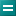Equal Tests whether the two input parameters are equal.Greater Than Tests whether the first input parameter is greater than the second input parameter.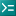Greater Than or Equal To Tests whether the first input parameter is greater than or equal to the second input parameter.IsNil Test whether the input parameter is Nil.Less Than Tests whether the first input parameter is less than the second input parameter.Less Than or Equal To Tests whether the first input parameter is less than or equal to the second input parameter.Logical AND Determines whether all of the specified input parameters are true.Logical Date Determines whether the input parameter is a date.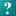Logical Existence Determines whether the record or field that is linked to it exists in a particular source instance message.Logical NOT Returns the logical inverse of the input parameter.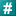Logical Numeric Determines whether the input parameter is a numeric value.Logical OR Determines whether any of the specified input parameters are true.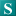Logical String Determines whether the input parameter is a string.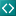Not Equal Tests whether the two input parameters are not equal.